# Thread: Samurai, Without Ever Having Felt Sorry For Itself

1. ## April, 2021

2021/4/4 (Sun) 45min

Bench Press
110 lbs (50kg) x 10
132 lbs (60kg) x 6
143 lbs (65kg) x 6
154 lbs (70kg) x 6
165 lbs (75kg) x 6
176 lbs (80kg) x 6
187 lbs (85kg) x 6
192 lbs (87kg) x 5
187 lbs (85kg) x 6
Total Reps 57

Weighted Chin up
BW (0kg) x 10
11 lbs (5kg) x 10
22 lbs (10kg) x 6
33 lbs (15kg) x 6
44 lbs (20kg) x 6
55 lbs (25kg) x 6
66 lbs (30kg) x 6
66 lbs (30kg) x 7
66 lbs (30kg) x 8
66 lbs (30kg) x 8
55 lbs (25kg) x 8
Total Reps 81

2021/4/8 (Thu) 43min

Bench Press
110 lbs (50kg) x 10
132 lbs (60kg) x 10
154 lbs (70kg) x 10
176 lbs (80kg) x 10
176 lbs (80kg) x 8
176 lbs (80kg) x 8
176 lbs (80kg) x 5
Total Reps 61

Weighted Chin up
BW (0kg) x 10
11 lbs (5kg) x 10
22 lbs (10kg) x 10
33 lbs (15kg) x 10
44 lbs (20kg) x 10
33 lbs (15kg) x 10
22 lbs (10kg) x 15
11 lbs (5kg) x 15
BW (0kg) x 15 *pull-ups
Total Reps 105

2021/4/11 (Sun) 30min

Bench Press
110 lbs (50kg) x 10
132 lbs (60kg) x 10
154 lbs (70kg) x 10
176 lbs (80kg) x 10
176 lbs (80kg) x 8
Total Reps 48

Weighted Chin up
BW (0kg) x 10
11 lbs (5kg) x 10
22 lbs (10kg) x 10
33 lbs (15kg) x 10
44 lbs (20kg) x 10
55 lbs (25kg) x 10
Total Reps 60

2021/4/14 (Wed) 46min

Bench Press
110 lbs (50kg) x 10
132 lbs (60kg) x 10
154 lbs (70kg) x 6
165 lbs (75kg) x 6
176 lbs (80kg) x 6
187 lbs (85kg) x 6
187 lbs (85kg) x 7
187 lbs (85kg) x 5
176 lbs (80kg) x 6 *partial
154 lbs (70kg) x 10 *partial
Total Reps 72

Weighted Chin up
BW (0kg) x 10
11 lbs (5kg) x 10
22 lbs (10kg) x 10
33 lbs (15kg) x 10
44 lbs (20kg) x 6
55 lbs (25kg) x 6
66 lbs (30kg) x 6
66 lbs (30kg) x 6
66 lbs (30kg) x 6
55 lbs (25kg) x 10
BW (0kg) x 20 *pull-ups
Total Reps 100

2021/4/18 (Sun) 37min

Bench Press
110 lbs (50kg) x 10
132 lbs (60kg) x 10
154 lbs (70kg) x 10
179 lbs (81kg) x 11 *9+2
179 lbs (81kg) x 8
165 lbs (75kg) x 8 *Partial
154 lbs (70kg) x 8 *Partial
Total Reps 65

Weighted Chin up
BW (0kg) x 10
11 lbs (5kg) x 10
22 lbs (10kg) x 10
33 lbs (15kg) x 10
44 lbs (20kg) x 10
55 lbs (25kg) x 6
66 lbs (30kg) x 6
66 lbs (30kg) x 6
55 lbs (25kg) x 6
BW (0kg) x 15 *Pull-ups
Total Reps 89

2021/4/21 (Wed) 36min

Bench Press
110 lbs (50kg) x 10
132 lbs (60kg) x 10
154 lbs (70kg) x 10
157 lbs (71kg) x 10
159 lbs (72kg) x 10
161 lbs (73kg) x 10
154 lbs (70kg) x 10 *Partial
154 lbs (70kg) x 8 *Partial
Total Reps 78

Weighted Chin up
BW (0kg) x 10
BW (0kg) x 10
BW (0kg) x 10
BW (0kg) x 10
BW (0kg) x 10
BW (0kg) x 10
BW (0kg) x 10
BW (0kg) x 15
BW (0kg) x 15 *Pull-ups
Total Reps 100

2021/4/25 (Sun) 55min

Bench Press
110 lbs (50kg) x 10
132 lbs (60kg) x 10
154 lbs (70kg) x 6
165 lbs (75kg) x 6
176 lbs (80kg) x 6
187 lbs (85kg) x 6
198 lbs (90kg) x 4
209 lbs (95kg) x 2
216 lbs (98kg) x 1
220 lbs (100kg) x 1
220 lbs (100kg) x 1
209 lbs (95kg) x 2
198 lbs (90kg) x 5
176 lbs (80kg) x 6 *partial
Total Reps 66

Weighted Chin up
BW (0kg) x 10
11 lbs (5kg) x 10
22 lbs (10kg) x 6
33 lbs (15kg) x 6
44 lbs (20kg) x 6
55 lbs (25kg) x 6
66 lbs (30kg) x 6
66 lbs (30kg) x 6
55 lbs (25kg) x 6
44 lbs (20kg) x 10
33 lbs (15kg) x 10
22 lbs (10kg) x 10
BW (0kg) x 20 *Pull-ups
Total Reps 112

2021/4/29 (Thu) 60min

Bench Press
110 lbs (50kg) x 10
132 lbs (60kg) x 10
154 lbs (70kg) x 6
170 lbs (77kg) x 6
181 lbs (82kg) x 11 *9+2
183 lbs (83kg) x 10 *8+2
176 lbs (80kg) x 6 *partial
176 lbs (80kg) x 8
154 lbs (70kg) x 10
Total Reps 77

Weighted Chin up
BW (0kg) x 10
11 lbs (5kg) x 10
22 lbs (10kg) x 10
33 lbs (15kg) x 10
44 lbs (20kg) x 10
55 lbs (25kg) x 6
66 lbs (30kg) x 6
77 lbs (35kg) x 5
66 lbs (30kg) x 6
55 lbs (25kg) x 8
BW (0kg) x 20
Total Reps 101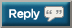2. April 2021CIMG6875.JPG
CIMG7063.JPG3. April 2021 (II)CIMG7154.jpg
CIMG7228.jpg4. ## May, 2021

2021/5/2 (Sun) 38min

Bench Press
110 lbs (50kg) x 10
132 lbs (60kg) x 10
154 lbs (70kg) x 8
165 lbs (75kg) x 6
176 lbs (80kg) x 6
187 lbs (85kg) x 8 *6+2
176 lbs (80kg) x 8 *partial
Total Reps 56

Weighted Chin up
BW (0kg) x 10
BW (0kg) x 10
BW (0kg) x 10
11 lbs (5kg) x 10
11 lbs (5kg) x 10
11 lbs (5kg) x 10
22 lbs (10kg) x 10
22 lbs (10kg) x 10
BW (0kg) x 20 *Pullups
Total Reps 100

2021/5/5 (Wed) 70min

Bench Press
110 lbs (50kg) x 10
132 lbs (60kg) x 10
154 lbs (70kg) x 10
176 lbs (80kg) x 6
187 lbs (85kg) x 6
198 lbs (90kg) x 3
209 lbs (95kg) x 2
216 lbs (98kg) x 1
220 lbs (100kg) x 1
220 lbs (100kg) x 1
209 lbs (95kg) x 2
198 lbs (90kg) x 4
187 lbs (85kg) x 6
Total Reps 62

Weighted Chin up
BW (0kg) x 10
11 lbs (5kg) x 10
22 lbs (10kg) x 10
33 lbs (15kg) x 10
44 lbs (20kg) x 10
55 lbs (25kg) x 10
66 lbs (30kg) x 6
66 lbs (30kg) x 6
55 lbs (25kg) x 10
44 lbs (20kg) x 10
33 lbs (15kg) x 10
Total Reps 102

2021/5/9 (Sun) 46min

Bench Press
110 lbs (50kg) x 10
132 lbs (60kg) x 10
157 lbs (71kg) x 10
159 lbs (72kg) x 10
161 lbs (73kg) x 10
163 lbs (74kg) x 10
165 lbs (75kg) x 8
154 lbs (70kg) x 8 *partial
Total Reps 76

Weighted Chin up
BW (0kg) x 10
BW (0kg) x 10
BW (0kg) x 10
11 lbs (5kg) x 10
11 lbs (5kg) x 10
11 lbs (5kg) x 10
22 lbs (10kg) x 10
22 lbs (10kg) x 10
22 lbs (10kg) x 10
BW (0kg) x 10 *Pullups
Total Reps 100

2021/5/12 (Wed) 59min

Bench Press
110 lbs (50kg) x 5
121 lbs (55kg) x 5
132 lbs (60kg) x 6
143 lbs (65kg) x 6
154 lbs (70kg) x 6
165 lbs (75kg) x 6
176 lbs (80kg) x 6
187 lbs (85kg) x 6
198 lbs (90kg) x 5
187 lbs (85kg) x 5
176 lbs (80kg) x 8 *Partial
Total Reps 64

Weighted Chin up
BW (0kg) x 10 + next set
11 lbs (5kg) x 5
22 lbs (10kg) x 5 + next set
33 lbs (15kg) x 5
44 lbs (20kg) x 5 + next set
55 lbs (25kg) x 5
66 lbs (30kg) x 6
66 lbs (30kg) x 7
66 lbs (30kg) x 7
66 lbs (30kg) x 8
66 lbs (30kg) x 8
55 lbs (25kg) x 10
44 lbs (20kg) x 10
33 lbs (15kg) x 10 *Pull-ups
Total Reps 101

2021/5/15 (Sat) 49min

Bench Press
110 lbs (50kg) x 10
132 lbs (60kg) x 10
154 lbs (70kg) x 10
176 lbs (80kg) x 10
165 lbs (75kg) x 10
154 lbs (70kg) x 10
154 lbs (70kg) x 11
154 lbs (70kg) x 10
176 lbs (80kg) x 6 *Partial
Total Reps 87

Weighted Chin up
BW (0kg) x 10
BW (0kg) x 10
BW (0kg) x 10
11 lbs (5kg) x 10
11 lbs (5kg) x 10
11 lbs (5kg) x 10
22 lbs (10kg) x 10
22 lbs (10kg) x 10
22 lbs (10kg) x 10
BW (0kg) x 15 *Pullups
Total Reps 105

2021/5/19 (Wed) 64min

Bench Press
110 lbs (50kg) x 10
132 lbs (60kg) x 10
154 lbs (70kg) x 6
165 lbs (75kg) x 6
176 lbs (80kg) x 6
187 lbs (85kg) x 6
198 lbs (90kg) x 4
209 lbs (95kg) x 2
216 lbs (98kg) x 1
220 lbs (100kg) x 1
220 lbs (100kg) x 1
216 lbs (98kg) x 1
209 lbs (95kg) x 2
198 lbs (90kg) x 5
176 lbs (80kg) x 8 *Partial
Total Reps 69

Weighted Chin up
BW (0kg) x 10
11 lbs (5kg) x 10
22 lbs (10kg) x 10
33 lbs (15kg) x 6
44 lbs (20kg) x 6
55 lbs (25kg) x 6
66 lbs (30kg) x 6
66 lbs (30kg) x 6
77 lbs (35kg) x 6
77 lbs (35kg) x 6
66 lbs (30kg) x 10
55 lbs (25kg) x 10
44 lbs (20kg) x 10
33 lbs (15kg) x 10
Total Reps 112

2021/5/22 (Sat) 39min

Bench Press
110 lbs (50kg) x 10
132 lbs (60kg) x 10
154 lbs (70kg) x 10
176 lbs (80kg) x 10
176 lbs (80kg) x 5
176 lbs (80kg) x 6
176 lbs (80kg) x 8 *Partial
Total Reps 59

Weighted Chin up
BW (0kg) x 10
BW (0kg) x 10
BW (0kg) x 10
BW (0kg) x 10
BW (0kg) x 10
BW (0kg) x 10
BW (0kg) x 10
BW (0kg) x 10
BW (0kg) x 10
BW (0kg) x 15 *Pullups
Total Reps 105

2021/5/25 (Tue) 56min

Bench Press
110 lbs (50kg) x 10
132 lbs (60kg) x 6
143 lbs (65kg) x 6
154 lbs (70kg) x 6
165 lbs (75kg) x 6
176 lbs (80kg) x 6
187 lbs (85kg) x 6
198 lbs (90kg) x 5
198 lbs (90kg) x 3
187 lbs (85kg) x 6
176 lbs (80kg) x 7 *Partial
Total Reps 67

Weighted Chin up
BW (0kg) x 10
11 lbs (5kg) x 6
22 lbs (10kg) x 6
33 lbs (15kg) x 6
44 lbs (20kg) x 6
55 lbs (25kg) x 6
66 lbs (30kg) x 6
66 lbs (30kg) x 8
66 lbs (30kg) x 8
66 lbs (30kg) x 8
66 lbs (30kg) x 8
55 lbs (25kg) x 10
44 lbs (20kg) x 12
Total Reps 100

2021/5/29 (Sat) 40min

Bench Press
110 lbs (50kg) x 10
132 lbs (60kg) x 10
154 lbs (70kg) x 10
176 lbs (80kg) x 6
187 lbs (85kg) x 6
176 lbs (80kg) x 8
165 lbs (75kg) x 8
165 lbs (75kg) x 8 *Partial
Total Reps 66

Weighted Chin up
BW (0kg) x 10
BW (0kg) x 10
BW (0kg) x 12
11 lbs (5kg) x 12
11 lbs (5kg) x 12
11 lbs (5kg) x 12
22 lbs (10kg) x 12
22 lbs (10kg) x 12
22 lbs (10kg) x 12
Total Reps 1045. Great work, some impressive pull up strength.6. Great work, some impressive pull up strength.
^^THIS!!!

BW + 66 lbs for 8 reps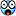Very strong, Kaz.7. ## June 2021

Great work, some impressive pull up strength.
^^THIS!!!

BW + 66 lbs for 8 repsVery strong, Kaz.
Thanks guys!

----------------

2021/6/1 (Tue) 69min

Bench Press
121 lbs (55kg) x 10
143 lbs (65kg) x 10
154 lbs (70kg) x 8
176 lbs (80kg) x 6
187 lbs (85kg) x 6
198 lbs (90kg) x 4
209 lbs (95kg) x 3
216 lbs (98kg) x 1
220 lbs (100kg) x 1
220 lbs (100kg) x 1
216 lbs (98kg) x 2
209 lbs (95kg) x 3
176 lbs (80kg) x 8 *Partial
Total Reps 63

Weighted Chin up
BW (0kg) x 10
11 lbs (5kg) x 10
22 lbs (10kg) x 10
33 lbs (15kg) x 6
44 lbs (20kg) x 6
55 lbs (25kg) x 6
66 lbs (30kg) x 6
66 lbs (30kg) x 8
66 lbs (30kg) x 8
66 lbs (30kg) x 6
55 lbs (25kg) x 10
44 lbs (20kg) x 10
BW (0kg) x 15 *Pullups
Total Reps 111

2021/6/5 (Sat) 44min

Bench Press
110 lbs (50kg) x 10
132 lbs (60kg) x 10
154 lbs (70kg) x 8
165 lbs (75kg) x 8
176 lbs (80kg) x 12
187 lbs (85kg) x 7
176 lbs (80kg) x 10
176 lbs (80kg) x 6 *Partial
Total Reps 71

Weighted Chin up
BW (0kg) x 10
BW (0kg) x 10
11 lbs (5kg) x 10
11 lbs (5kg) x 10
22 lbs (10kg) x 10
22 lbs (10kg) x 10
33 lbs (15kg) x 10
33 lbs (15kg) x 10
22 lbs (10kg) x 10
BW (0kg) x 15 *Partial
Total Reps 105

2021/6/9 (Wed) 61min

Bench Press
110 lbs (50kg) x 10
132 lbs (60kg) x 8
154 lbs (70kg) x 8
165 lbs (75kg) x 7
176 lbs (80kg) x 7
187 lbs (85kg) x 6
198 lbs (90kg) x 6
198 lbs (90kg) x 5
187 lbs (85kg) x 5
176 lbs (80kg) x 7 *Partial
Total Reps 69

Weighted Chin up
BW (0kg) x 10
11 lbs (5kg) x 10
22 lbs (10kg) x 10
33 lbs (15kg) x 6
44 lbs (20kg) x 6
55 lbs (25kg) x 10
66 lbs (30kg) x 6
77 lbs (35kg) x 6
77 lbs (35kg) x 6
66 lbs (30kg) x 8
55 lbs (25kg) x 10
44 lbs (20kg) x 12
Total Reps 88

2021/6/12 (Sat) 39min

Bench Press
110 lbs (50kg) x 15
110 lbs (50kg) x 15
110 lbs (50kg) x 15
110 lbs (50kg) x 15
110 lbs (50kg) x 15
110 lbs (50kg) x 20
110 lbs (50kg) x 15
Total Reps 110

Weighted Chin up
BW (0kg) x 15
BW (0kg) x 15
BW (0kg) x 15
BW (0kg) x 15
BW (0kg) x 15
BW (0kg) x 15
BW (0kg) x 20
BW (0kg) x 15
Total Reps 125

2021/6/15 (Tue) 72min

Bench Press
110 lbs (50kg) x 12
132 lbs (60kg) x 10
154 lbs (70kg) x 8
176 lbs (80kg) x 6
187 lbs (85kg) x 6
198 lbs (90kg) x 5
209 lbs (95kg) x 3
216 lbs (98kg) x 1
220 lbs (100kg) x 1
220 lbs (100kg) x 1
220 lbs (100kg) x 1
216 lbs (98kg) x 2
209 lbs (95kg) x 3
176 lbs (80kg) x 8 *Partial
Total Reps 67

Weighted Chin up
BW (0kg) x 10
11 lbs (5kg) x 10
22 lbs (10kg) x 10
33 lbs (15kg) x 10
44 lbs (20kg) x 10
55 lbs (25kg) x 10
66 lbs (30kg) x 6
66 lbs (30kg) x 6
66 lbs (30kg) x 10
66 lbs (30kg) x 8
55 lbs (25kg) x 10
44 lbs (20kg) x 10
Total Reps 110

2021/6/19 (Sat) 53min

Bench Press
110 lbs (50kg) x 10
132 lbs (60kg) x 10
154 lbs (70kg) x 10
176 lbs (80kg) x 8
187 lbs (85kg) x 5
190 lbs (86kg) x 5
192 lbs (87kg) x 5
187 lbs (85kg) x 7
176 lbs (80kg) x 8 *Partial
Total Reps 68

Weighted Chin up
BW (0kg) x 10
11 lbs (5kg) x 10
22 lbs (10kg) x 10
33 lbs (15kg) x 10
44 lbs (20kg) x 10
55 lbs (25kg) x 10
44 lbs (20kg) x 10
33 lbs (15kg) x 10
22 lbs (10kg) x 10
BW (0kg) x 10 *Pullups
Total Reps 100

2021/6/22 (Tue) 42min

Bench Press
110 lbs (50kg) x 10
132 lbs (60kg) x 10
154 lbs (70kg) x 8
176 lbs (80kg) x 12
187 lbs (85kg) x 8
176 lbs (80kg) x 9
176 lbs (80kg) x 6 *Partial
Total Reps 63

Weighted Chin up
BW (0kg) x 15
BW (0kg) x 15
BW (0kg) x 15
BW (0kg) x 15
11 lbs (5kg) x 15
11 lbs (5kg) x 15
11 lbs (5kg) x 15
BW (0kg) x 15 *Pull-ups
Total Reps 120

2021/6/25 (Fri) 70min

Bench Press
110 lbs (50kg) x 12
132 lbs (60kg) x 10
154 lbs (70kg) x 8
176 lbs (80kg) x 6
187 lbs (85kg) x 5
198 lbs (90kg) x 5
212 lbs (96kg) x 3
218 lbs (99kg) x 1
223 lbs (101kg) x 1
223 lbs (101kg) x 1
223 lbs (101kg) x 1
218 lbs (99kg) x 1
209 lbs (95kg) x 2
187 lbs (85kg) x 6 *Partial
Total Reps 62

Weighted Chin up
BW (0kg) x 10
11 lbs (5kg) x 10
22 lbs (10kg) x 10
33 lbs (15kg) x 6
44 lbs (20kg) x 6
55 lbs (25kg) x 8
66 lbs (30kg) x 8
66 lbs (30kg) x 8
66 lbs (30kg) x 8
66 lbs (30kg) x 8
55 lbs (25kg) x 10
44 lbs (20kg) x 10
Total Reps 102

2021/6/28 (Mon) 52min

Bench Press
110 lbs (50kg) x 10
132 lbs (60kg) x 10
154 lbs (70kg) x 5
165 lbs (75kg) x 5
176 lbs (80kg) x 5
187 lbs (85kg) x 5
187 lbs (85kg) x 5
187 lbs (85kg) x 5
187 lbs (85kg) x 5
176 lbs (80kg) x 7 *Partial
Total Reps 62

Weighted Chin up
BW (0kg) x 10
BW (0kg) x 10
BW (0kg) x 10
11 lbs (5kg) x 10
11 lbs (5kg) x 10
11 lbs (5kg) x 10
22 lbs (10kg) x 10
22 lbs (10kg) x 10
22 lbs (10kg) x 10
BW (0kg) x 10 *Pull-ups
Total Reps 1008. June 2021 (I)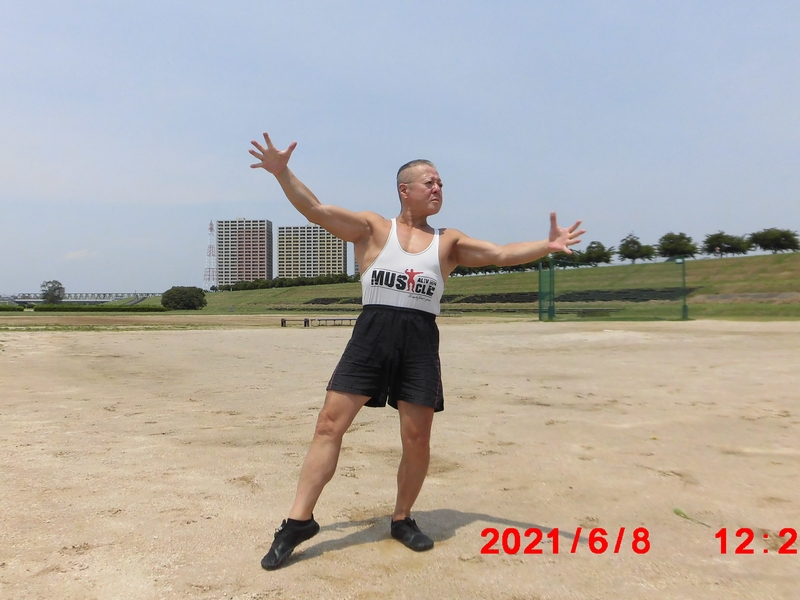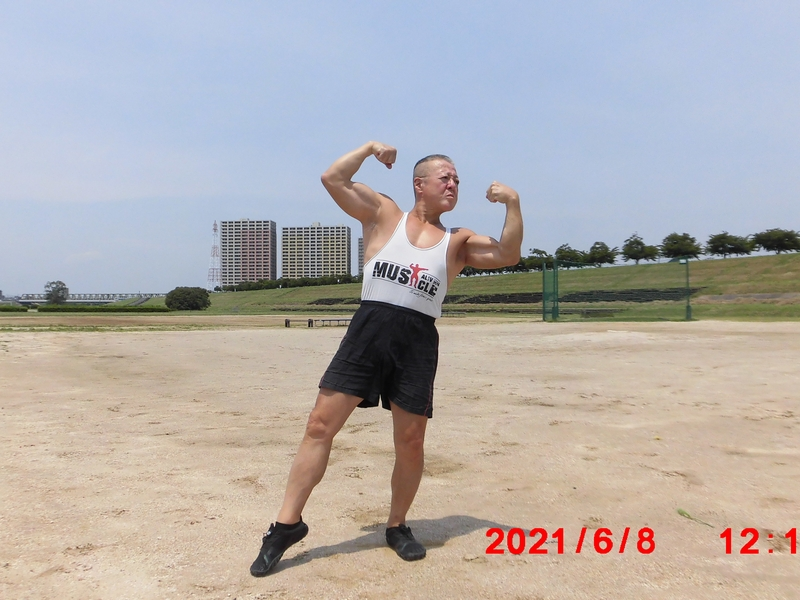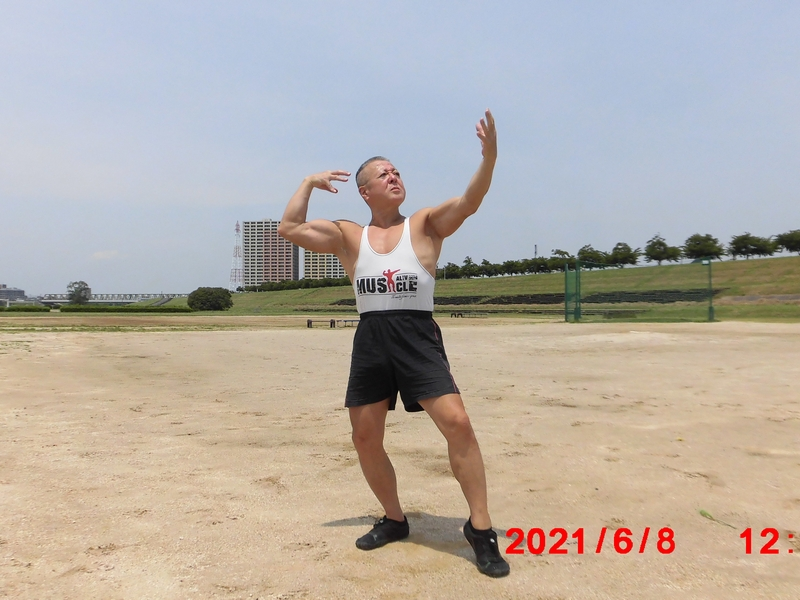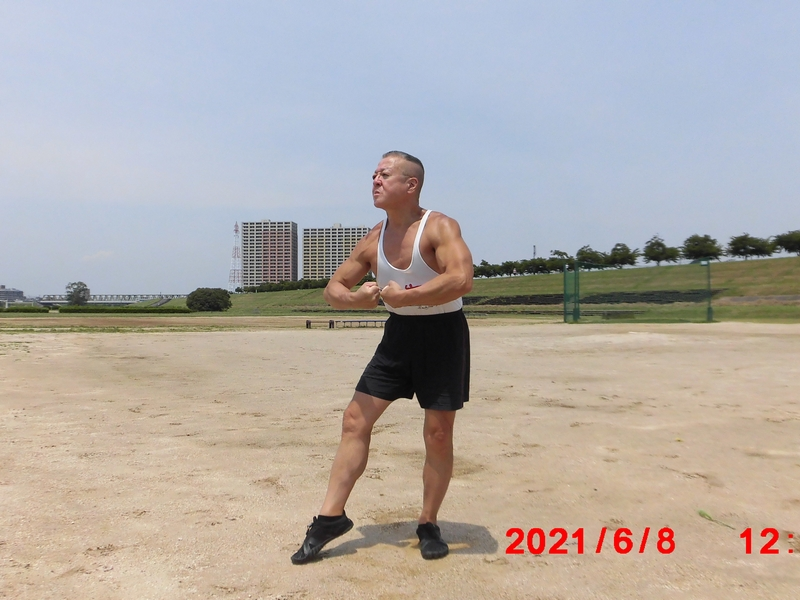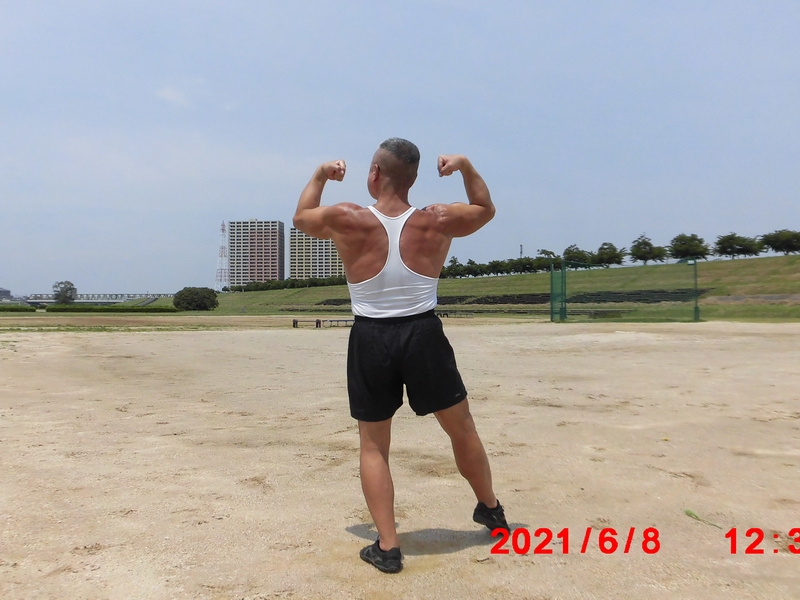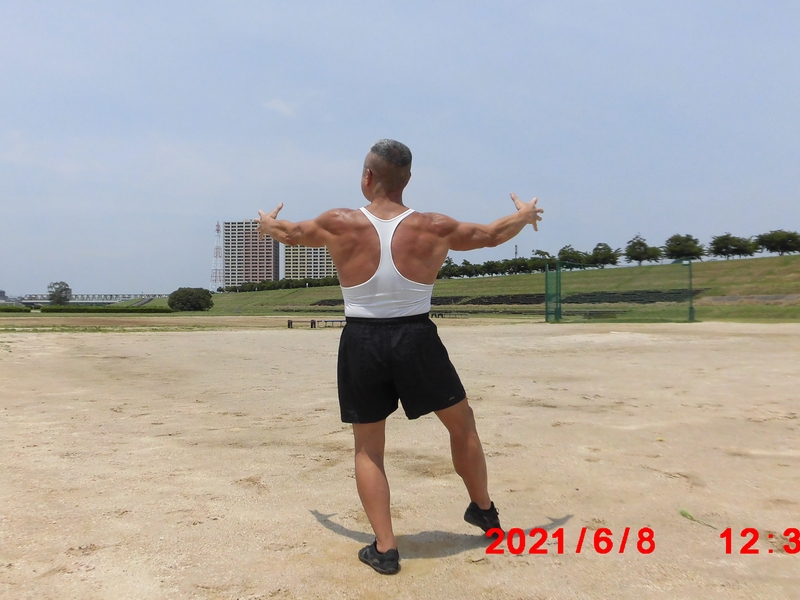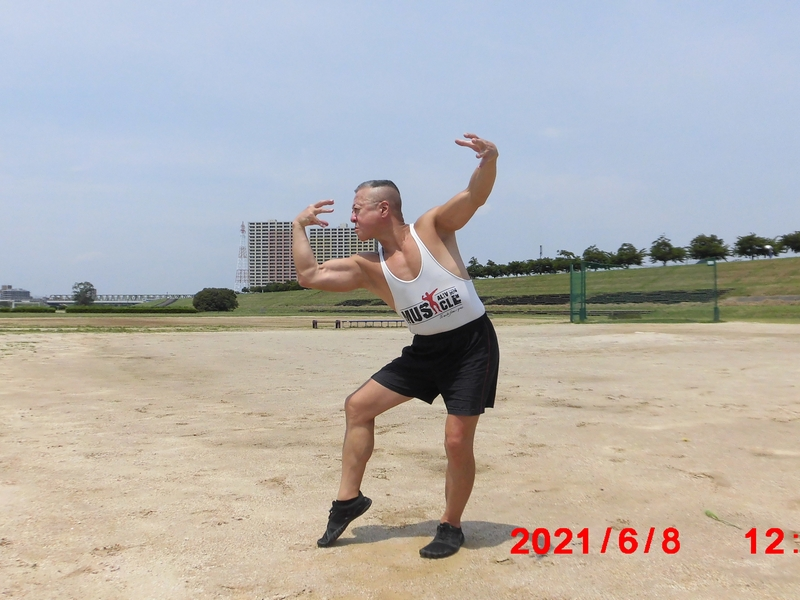9. June 2021 (II)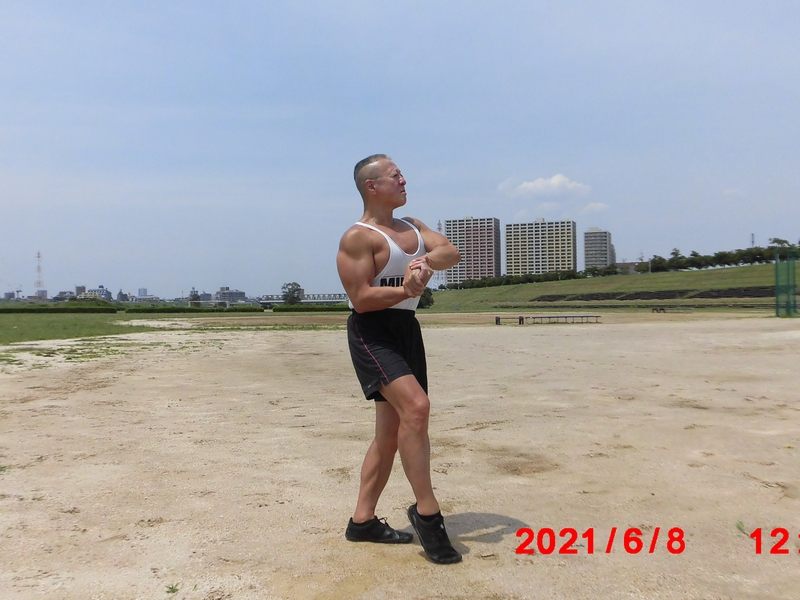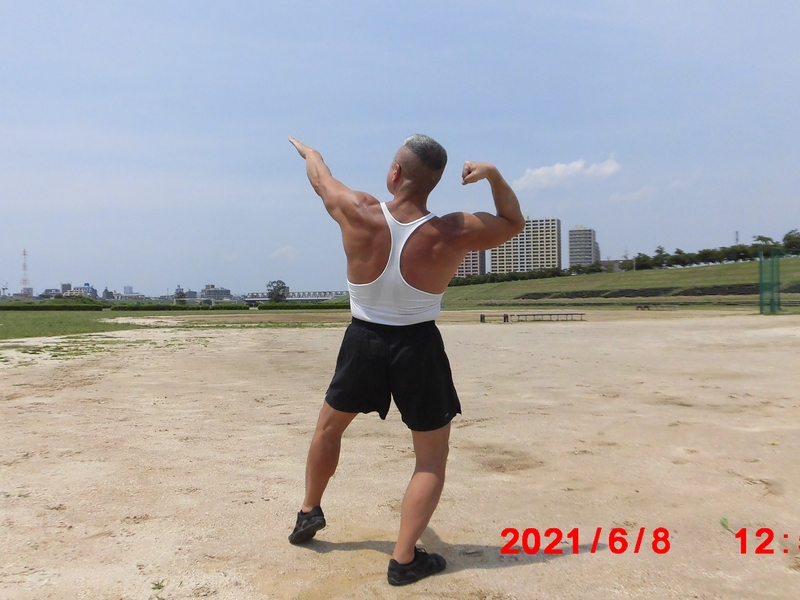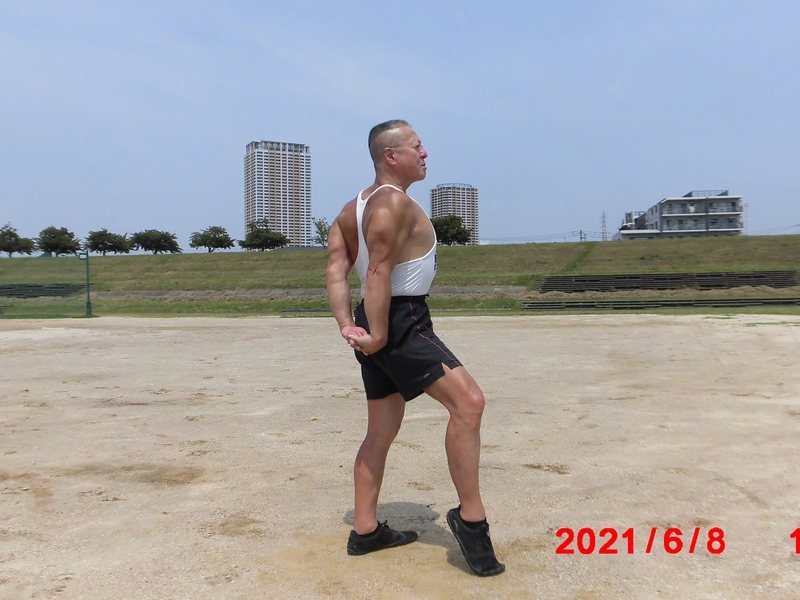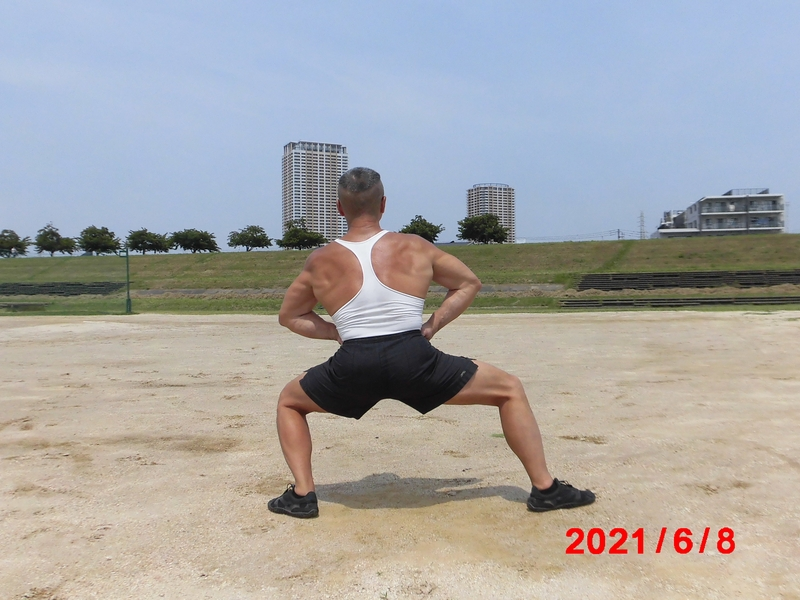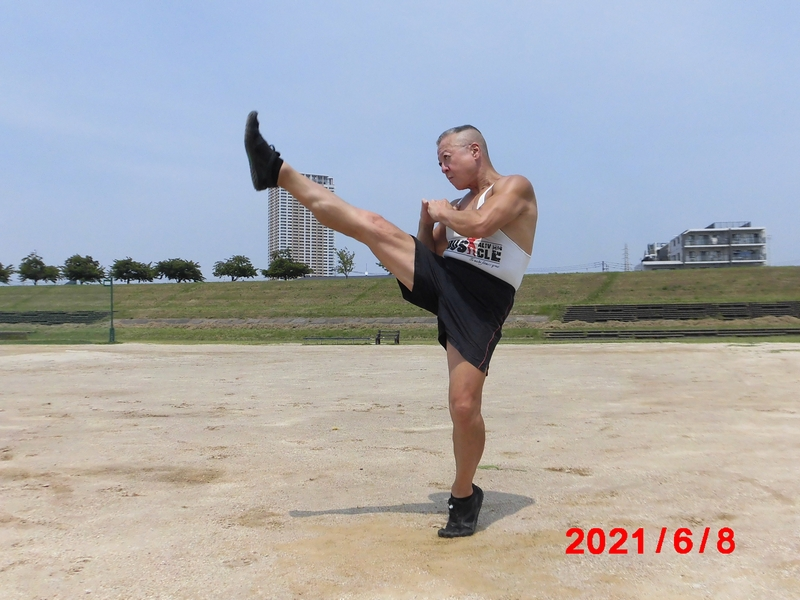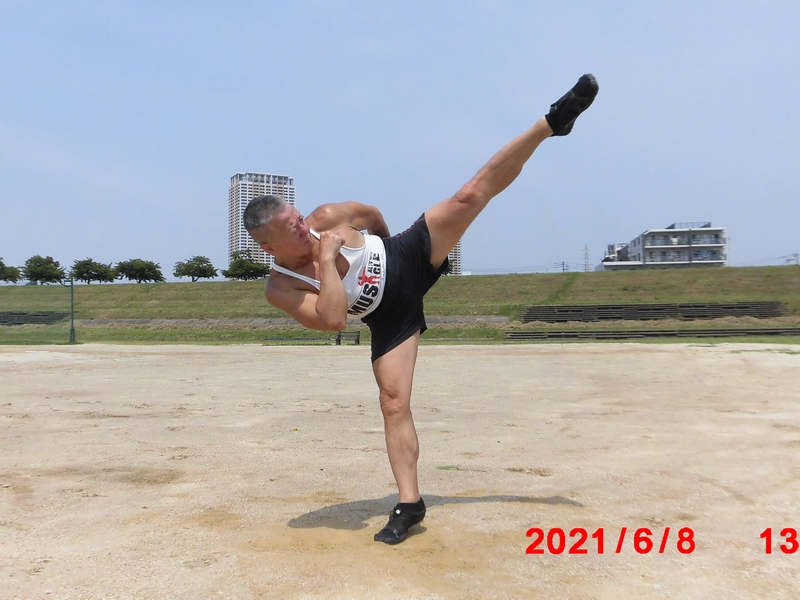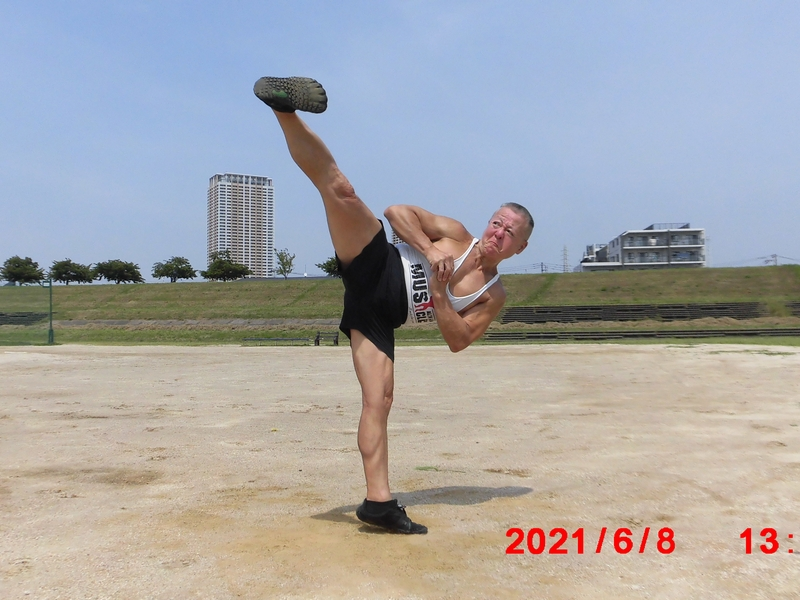10. Still my big brother and always my hero.Good to see you, Kaz.

xo
Athi#### Posting Permissions

• You may not post new threads
• You may not post replies
• You may not post attachments
• You may not edit your posts